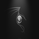# Indicator: Premier Stochastic Oscillator

19044 visualizzazioni
The PSO , developed by Lee Leibfarth, is a rewired version of a short-period stochastic . This provides a quick response to changes in market direction. This highly sensitive indicator allows for early anticipation of price turns and can be used to establish definitive trading zones that identify potential trading opportunities.

Rules as suggested by Mr.Lee:

(1) Premier stochastic crosses below 0.90
(2) premier stochastic crosses below 0.20

(1) Premier stochastic crosses above -0.90
(2) premier stochastic crosses above -0.20

http://www.investopedia.com/articles/tra...
```//
// @author LazyBear
//
study("Premier Stochastic Oscillator [LazyBear]", shorttitle="PSO_LB")
stochlen = input(8, title="Stoch length")
smoothlen = input(25, title="Smooth length")
sk = stoch( close, high, low, stochlen)
len = round(sqrt( smoothlen ))
nsk = 0.1 * ( sk - 50 )
ss = ema( ema( nsk, len ), len )
expss = exp( ss )
pso = ( expss - 1 )/( expss + 1 )
plot( pso, title="Premier Stoch", color=black, linewidth=2 )
plot( pso, color=iff( pso < 0, red, blue ), style=histogram )
plot(0, color=gray)
plot( 0.2, color=blue, style=3 )
plot( 0.9, color=blue)
plot( -0.2, color=red, style=3)
plot( -0.9, color=red )```
List of my free indicators: http://bit.ly/1LQaPK8
List of my indicators at Appstore: http://blog.tradingview.com/?p=970

## CommentiNot to second guess you on this - but the short/long trade parameters seem reversed from looking at the chart. It would seem when over 0.90 to go short, not go long... -0.90 go long, not short.